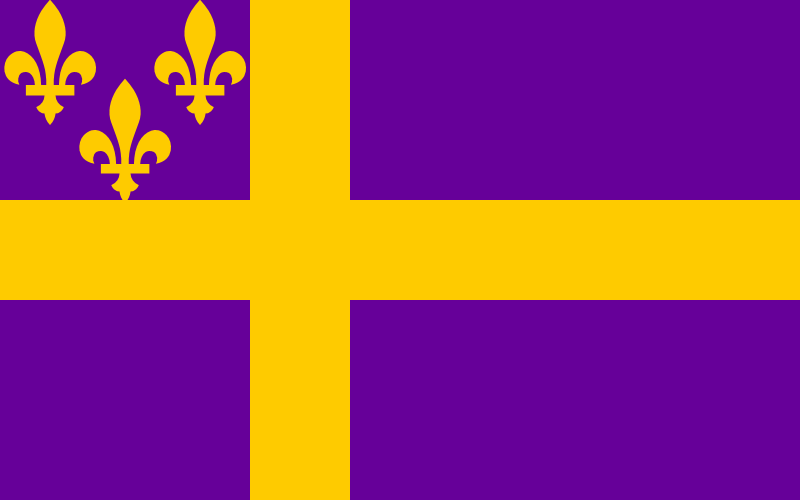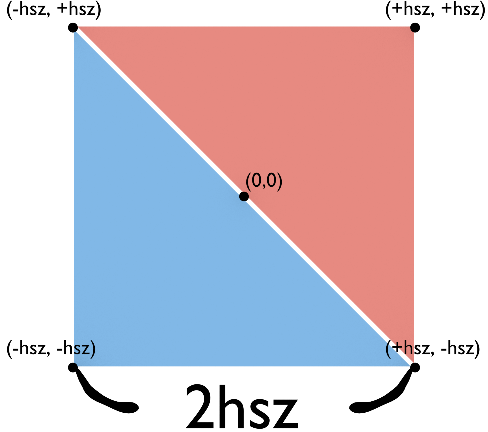# mid's siteyou're logged in as loser

## Journey into OpenGL: the Cube?

### The Series

I think a triangle is a rather boring shape when you consider what OpenGL is capable of. Here we're going to use our theoretical knowledge to build something more volumetric.

Welcome the Cube. The Cube, as you might know, is made of six squares. A square is made of two triangles, and those two triangles share 2 of 3 common vertices. As a triangle is made of three vertices, this means we must pass 36 vertices in order to get the full shape, when using `GL_TRIANGLES`.

Now while you can use `GL_QUADS` and push six quads (24 vertices) instead, I will be using triangles because they'll grow increasingly important anyway. A cube isn't really a real-world use case.Square on a plane.

``````static float hsz = 0.2; //Half-size

glBegin(GL_TRIANGLES);
glColor3f(1, 1, 1);

// Square on -Z plane
glVertex3f(-hsz, -hsz, -hsz);
glVertex3f(+hsz, -hsz, -hsz);
glVertex3f(-hsz, +hsz, -hsz);

glVertex3f(-hsz, +hsz, -hsz);
glVertex3f(+hsz, -hsz, -hsz);
glVertex3f(+hsz, +hsz, -hsz);

// On -X plane
glVertex3f(-hsz, -hsz, -hsz);
glVertex3f(-hsz, -hsz, +hsz);
glVertex3f(-hsz, +hsz, -hsz);

glVertex3f(-hsz, +hsz, -hsz);
glVertex3f(-hsz, -hsz, +hsz);
glVertex3f(-hsz, +hsz, +hsz);

// On -Y plane
glVertex3f(-hsz, -hsz, -hsz);
glVertex3f(+hsz, -hsz, -hsz);
glVertex3f(-hsz, -hsz, +hsz);

glVertex3f(-hsz, -hsz, +hsz);
glVertex3f(+hsz, -hsz, -hsz);
glVertex3f(+hsz, -hsz, +hsz);
glEnd();``````

Psych! I only gave you half the vertices, and the rest will be an exercise. Luckily, the vertices are well-organized, and you should be able to spot a pattern in them, leading you to solve it quickly. If you manage, you will reward yourself with a full cube. For now, have the half: